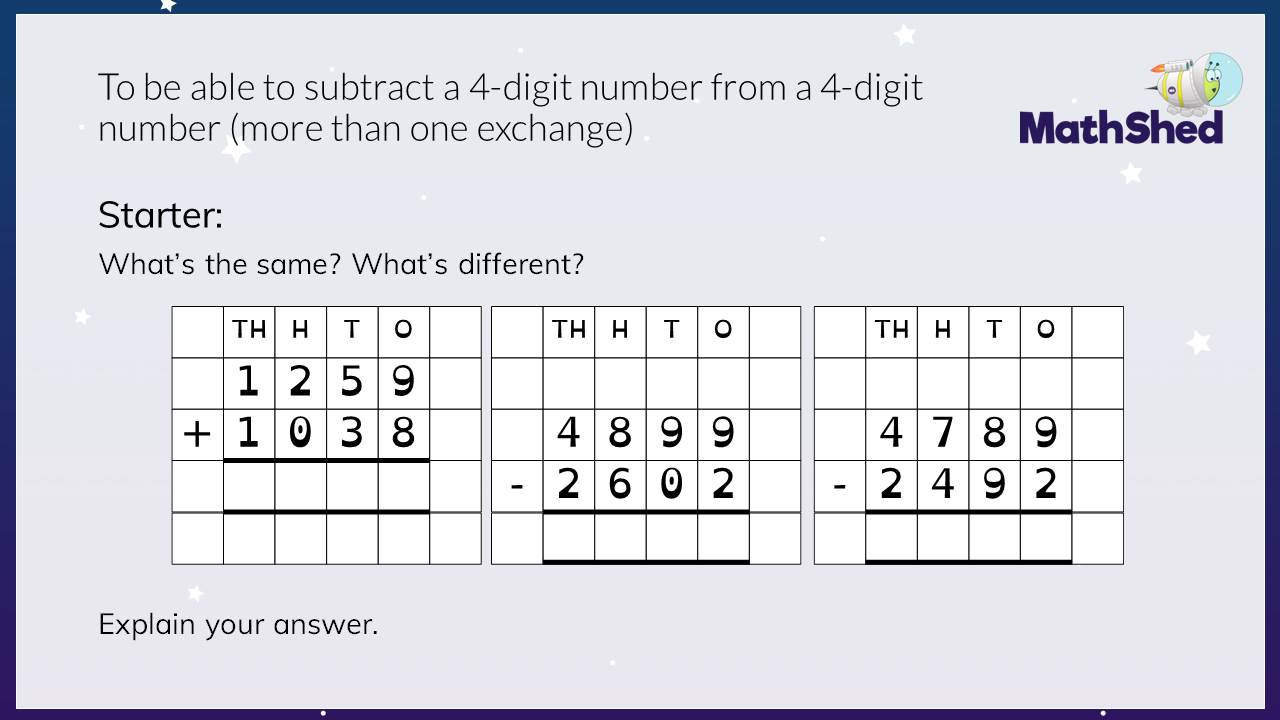Stage 4 - Term 1 - Block 2 - Addition and Subtraction - Lesson 7 - To be able to subtract a 4-digit number from a 4-digit number more than one exchangeStage 4 - Term 1 - Block 2 - Addition and Subtraction - Lesson 7 - To be able to subtract a 4-digit number from a 4-digit number more than one exchange